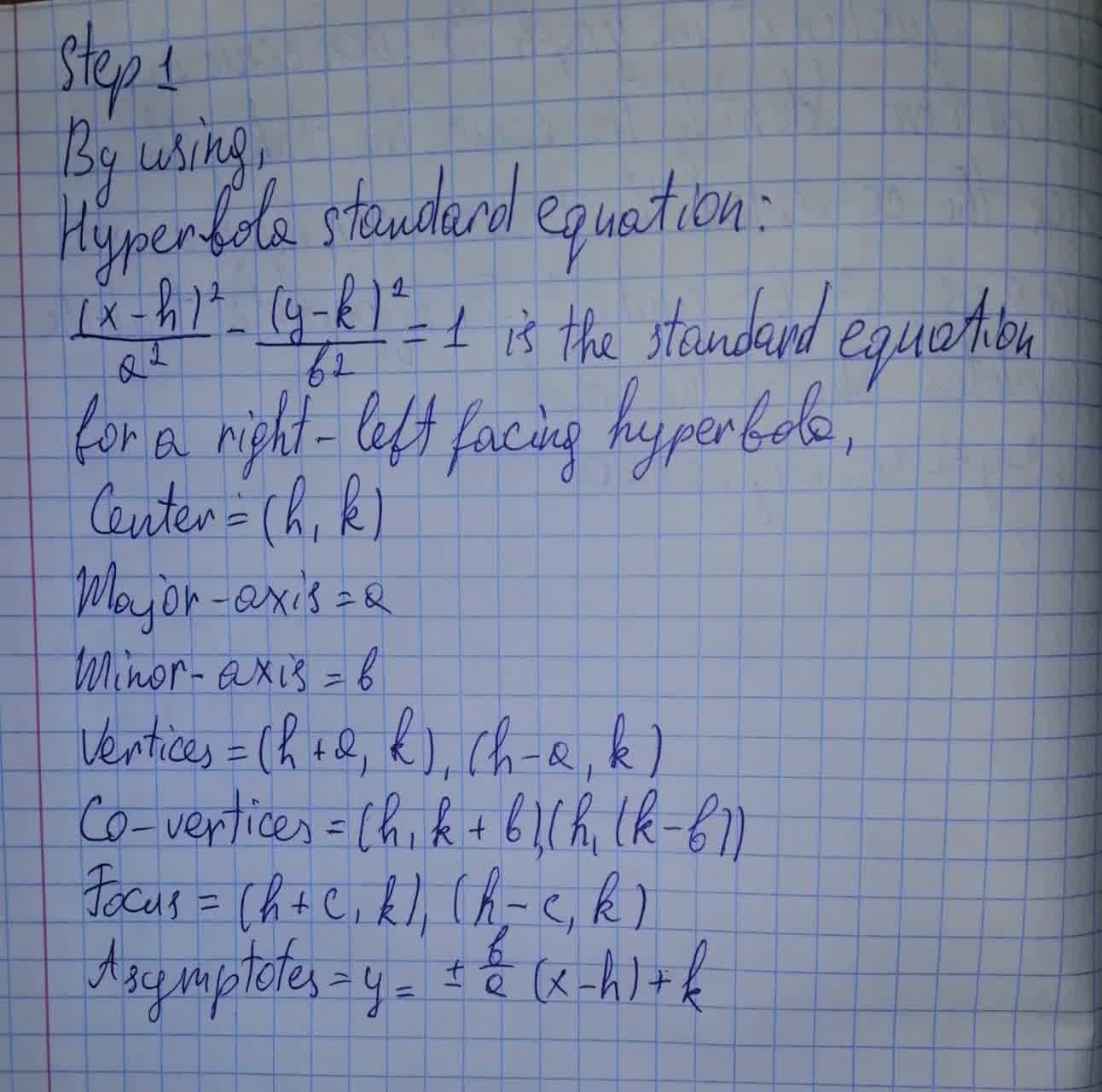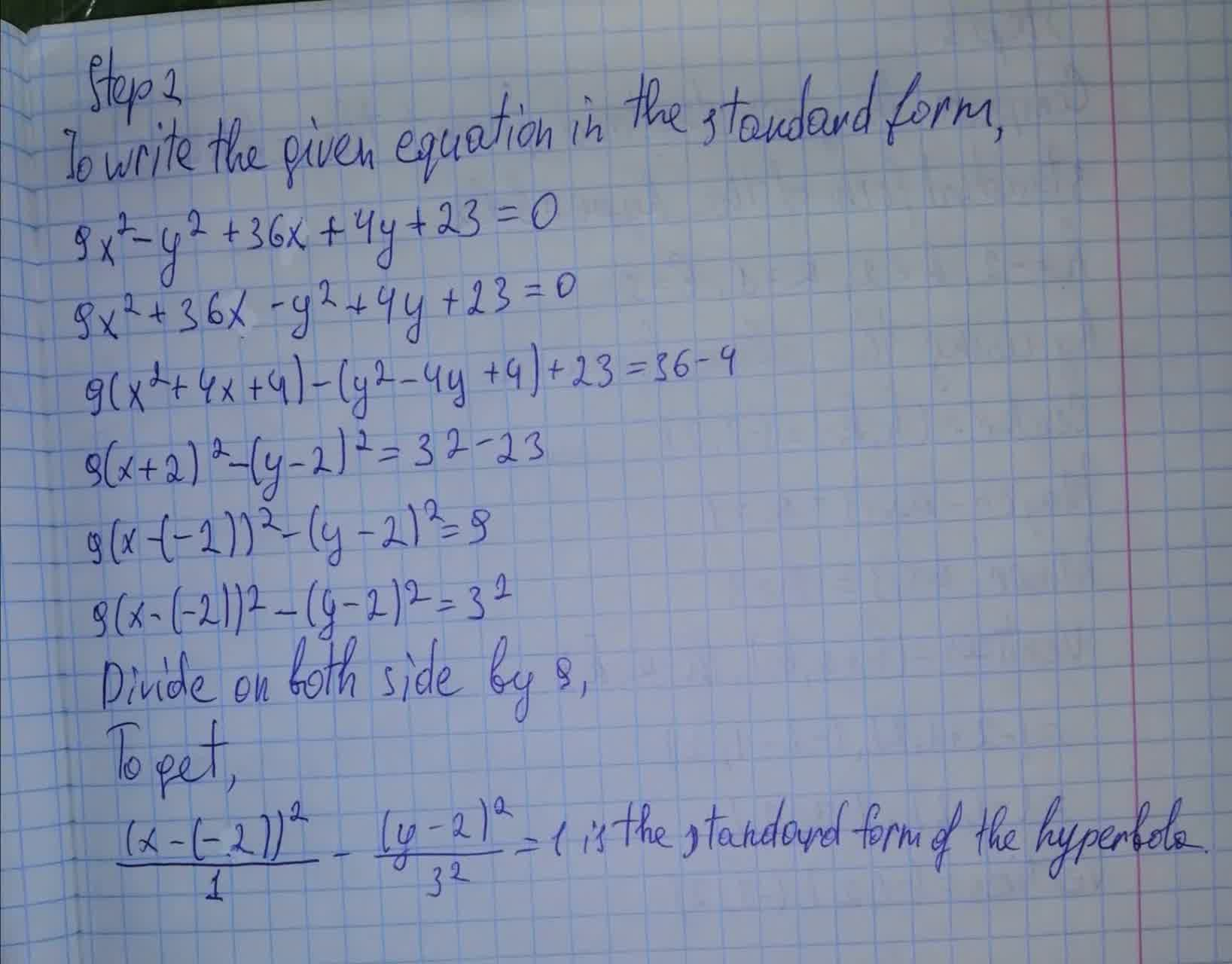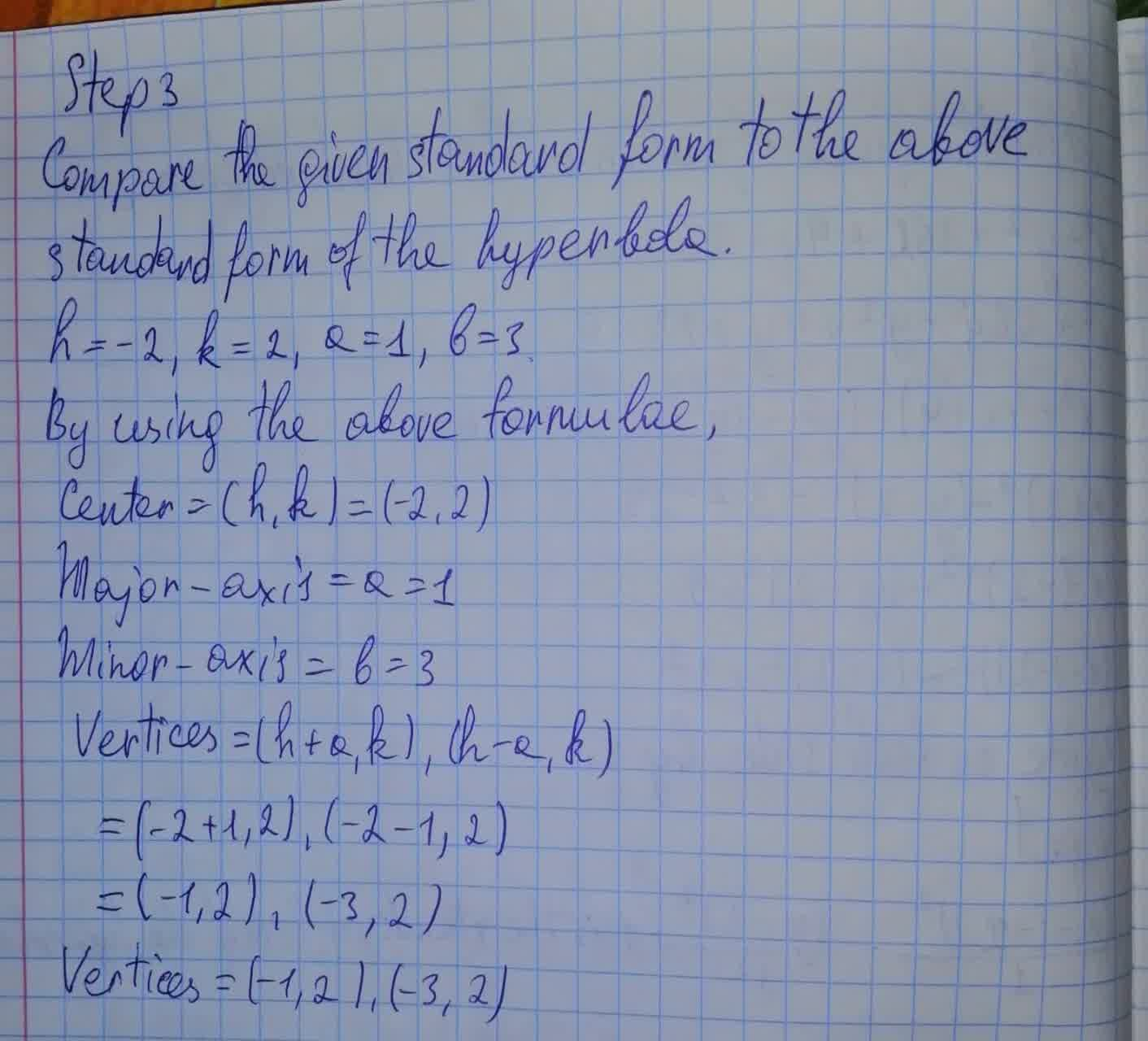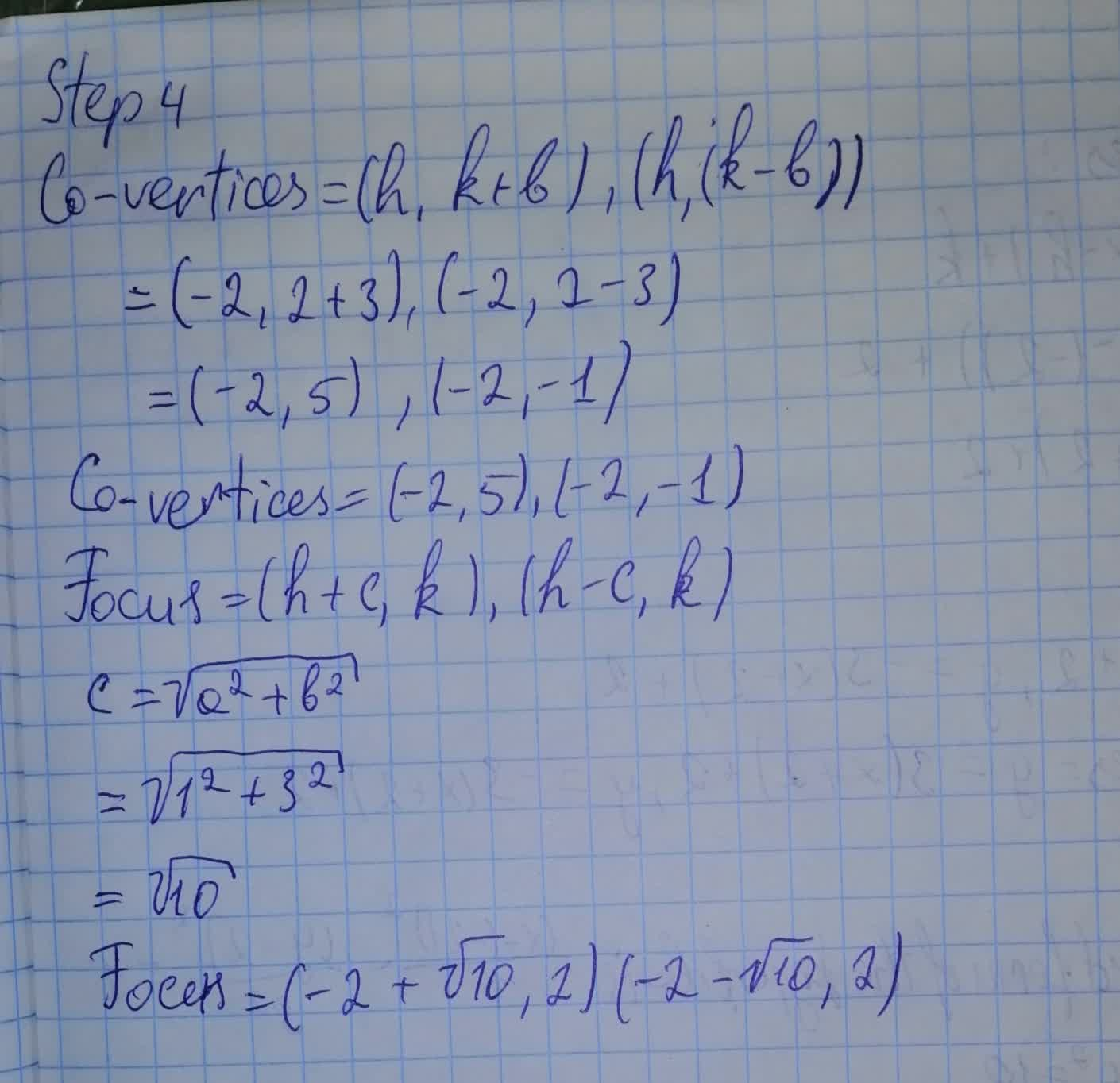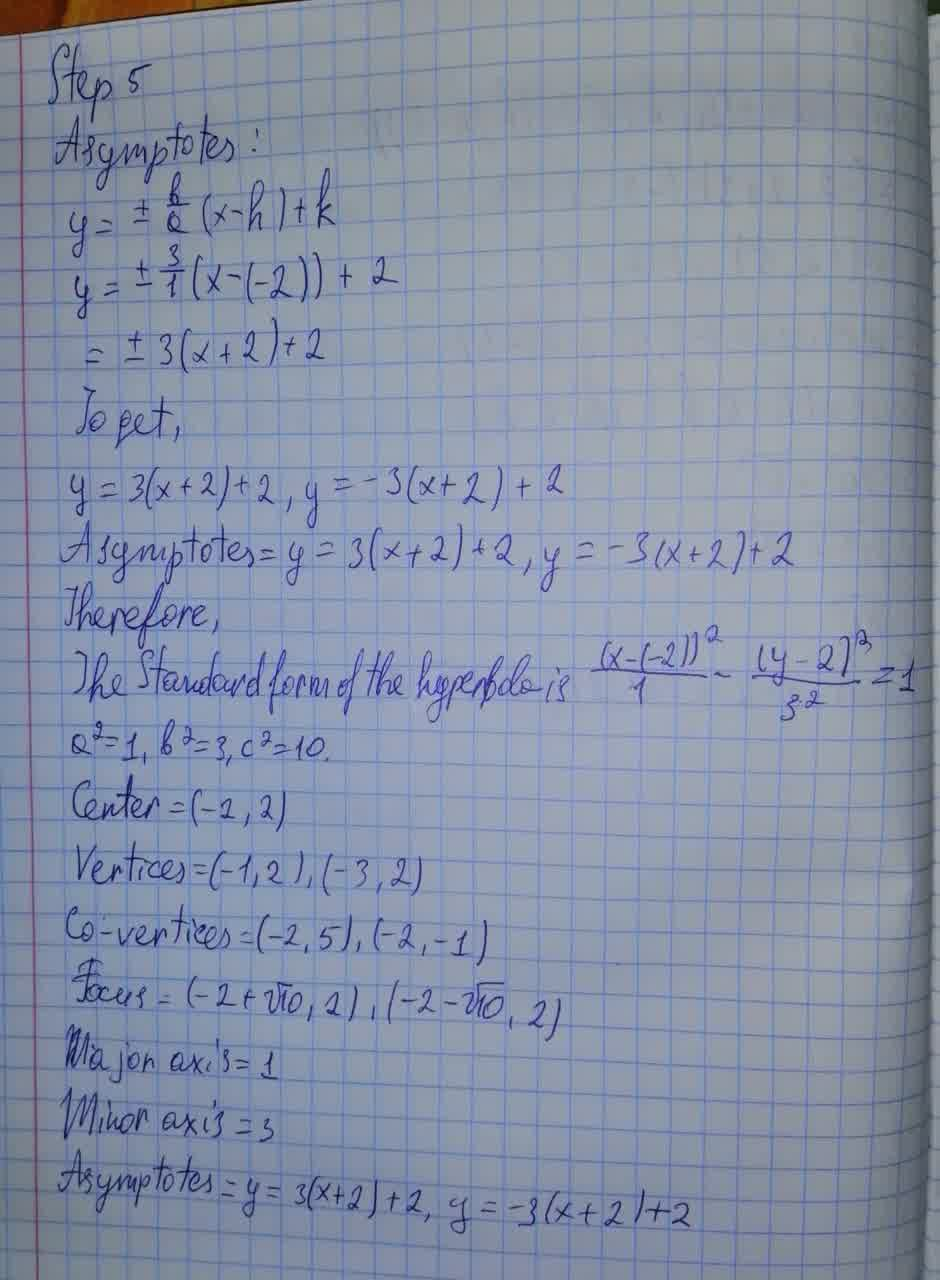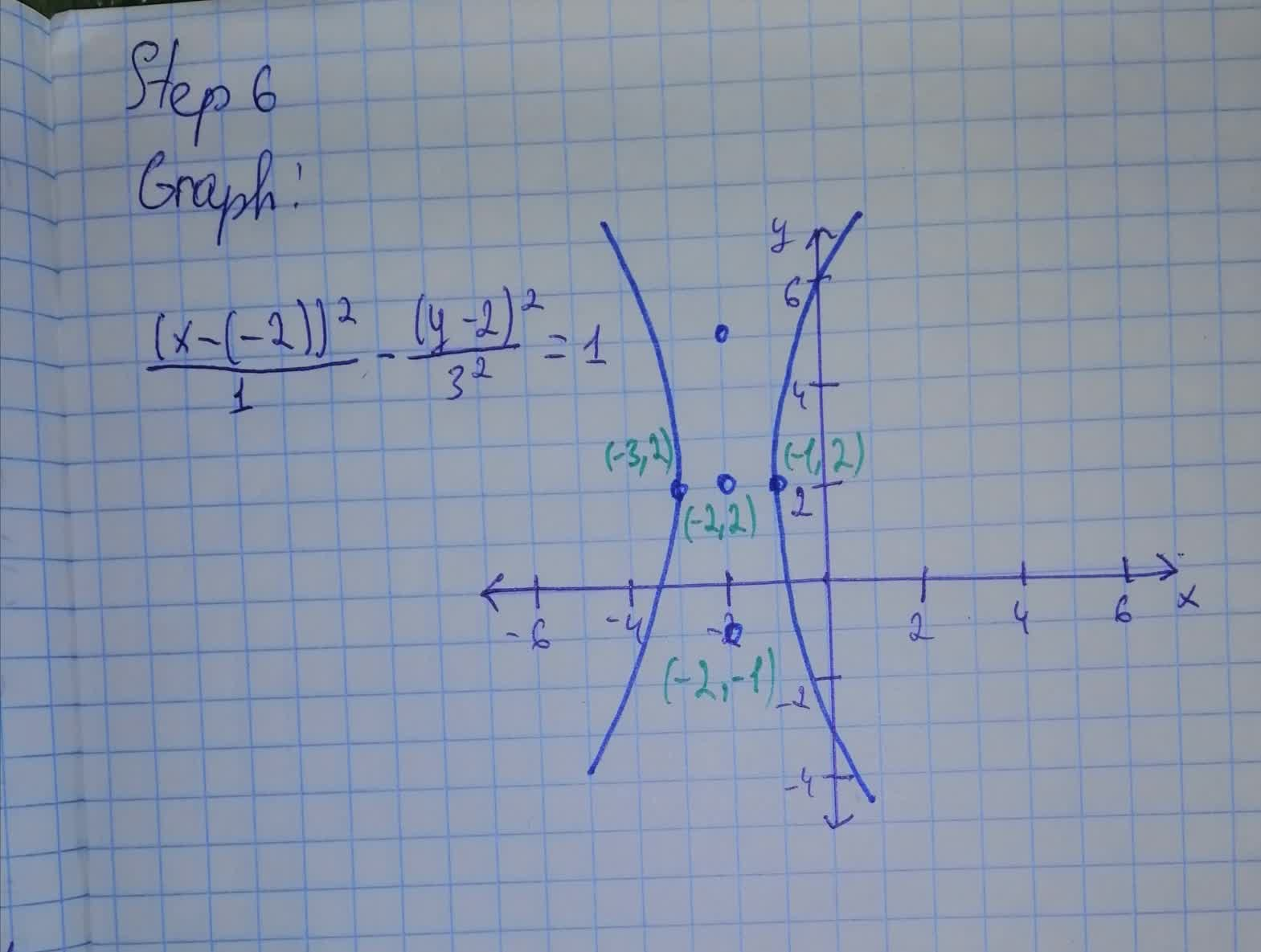Find the equation of the graph for each conic in standard form. Sketch the graph. 9x^2-y^2 + 36x+4y+23=0vestirme4 2021-08-07 Answered
Find the equation of the graph for each conic in standard form. Identify the conic, the center, the vertex, the co-vertex, the focus (foci), major axis, minor axis, $$\displaystyle{a}^{{2}},{b}^{{2}}$$, and $$\displaystyle{c}^{{2}}$$?. For hyperbola, find the asymptotes. Sketch the graph. $$\displaystyle{9}{x}^{{2}}-{y}^{{2}}+{36}{x}+{4}{y}+{23}={0}$$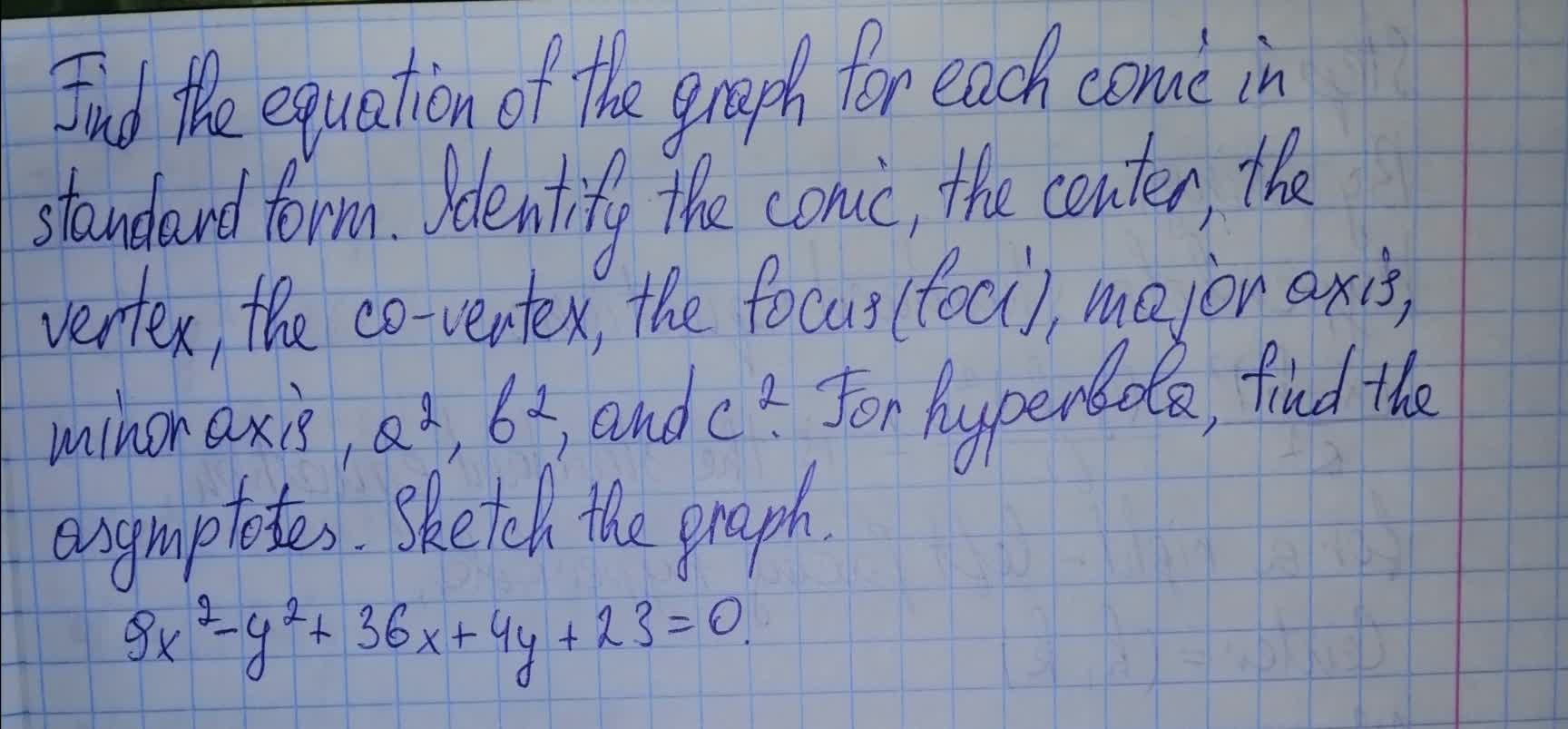• Questions are typically answered in as fast as 30 minutes

Solve your problem for the price of one coffee

• Math expert for every subject
• Pay only if we can solve itMacsen Nixon

Explanation: